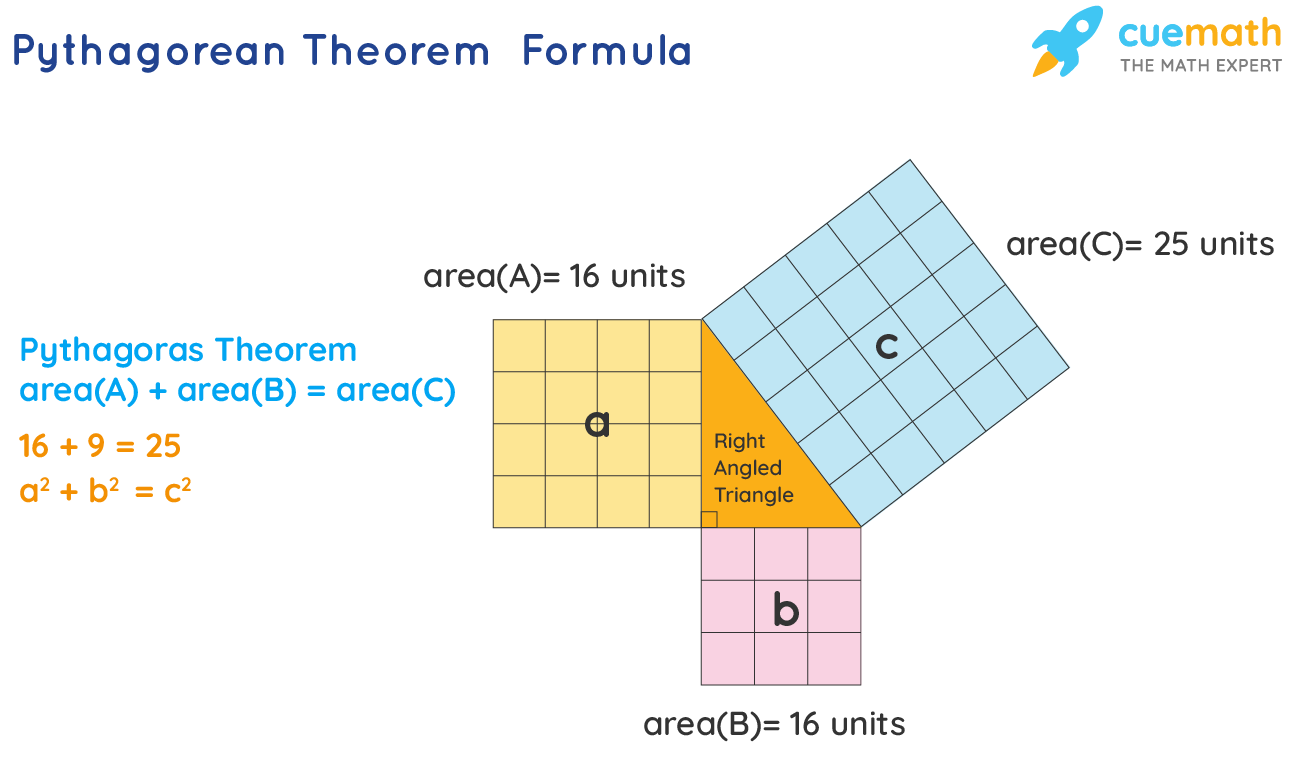# Pythagorean Theorem Formula

Pythagoras theorem formula was introduced by the Greek Mathematician Pythagoras of Samos. This theorem gives the fundamental aspect in Euclidean Geometry connecting the three sides of a triangle provided the triangle must be right-angled. Let's learn about the Pythagorean theorem formula with a few solved examples in the end.

## What Is the Pythagorean Theorem Formula?

As per the Pythagorean theorem formula, in a right-angled triangle, the square of the hypotenuse side is equal to the sum of the square of the other two sides (that is adjacent and opposite sides).## Solved Examples Using Pythagorean Theorem Formula

### Example 1:

A right-angled triangle ABC, has base BC = 12 cm, height AB = 5 cm. What is the length of AC?

Solution:

By Pythagoras Theorem we know that,
AB2 + BC2 = AC2
AC2 = 122 + 52
AC = (122 + 52)½ = (122 + 52)
AC = 13 cm

Answer: The length of AC is 13 cm.

### Example 2:

A right-angled triangle PQR, has angle Q = 90°. PQ = 8 cm. PR = 10 cm. Find QR.

Solution:

By Pythagoras Theorem we know that,
PQ2 + QR2 = PR2
102 = QR2 + 82
QR = (102 - 82)½ = √(102 - 82)
QR = 6 cm

Answer: The length of QR is 6 cm.